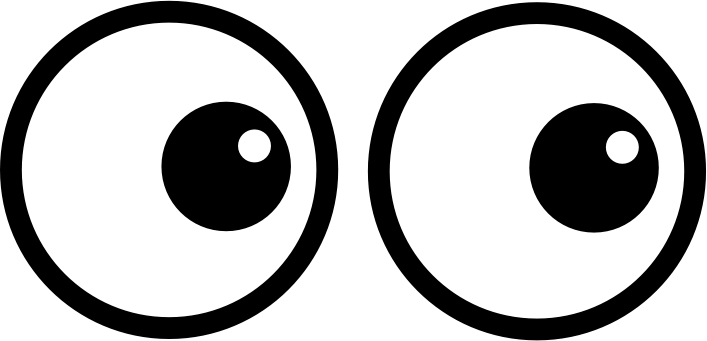# EXPS 1 | Lesson 3 | Try This! (Scientific Notation)Write the following numbers in scientific notation.

1. $$0.00234$$
2. $$0.00000543$$
3. $$459,000,000$$
4. $$67,000,000,000$$
5. $$0.0000401$$

Write the following numbers in standard notation.

1. $$3.87\times10^{-1}$$
2. $$4.01\times10^{6}$$
3. $$5.012\times10^{8}$$
4. $$1.21\times10^{-3}$$
5. $$5.87\times10^{-8}$$